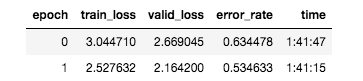# Clarifying Relationship Between Loss And Error Rate

I’m trying to get a better understanding of the connection between loss rate and error rate.In one of my training examples as you can see the loss rate went down, but the error rate also dropped by 10%.

In my mind, a lower loss rate would mean a better error rate, but here it’s not the case. Error rate I suppose is the objective accuracy (hot dog or not hot dog), whereas loss is the difference in the weights (so lower loss means the weights are closer to the correct answer). So even though my error rates are lower (meaning weights are closer to the correct answer) they’re still predicting the wrong label which is why the error rate is lower

^ Is that an accurate conceptual understanding of the relationship between the two or am I missing something?

Hi @andreilys pls look at this post whether this will answer your query Lesson 1 : Train_loss, valid_loss, error_rate